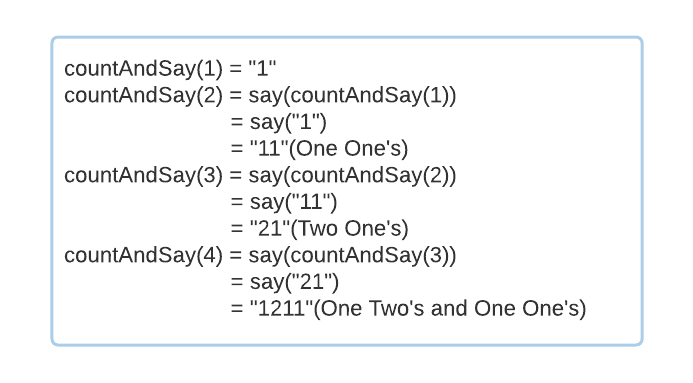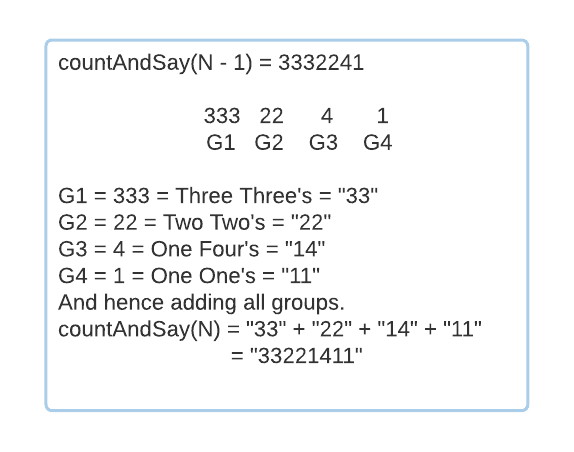# Count and Say

## Introduction

There is an algorithm for anything, from inverting a binary tree to proposing somebody. Although computer algorithms are not novels, people read them as if they are. However, they are mathematics written uniquely.

"An algorithm must be seen to be believed," said Sir Donald Knuth. The most straightforward approach to learning an algorithm is to write it instead of reading it. So grab a notepad and pen and join us in discussing the logic.

The problem we are going to discuss today is one with different approaches but the same math. Now let’s define the problem.

## Problem Statement

In this problem, you'll be given an integer 'N', and you have to return the count and say sequence for 'N'.

This count and say sequence can be best defined recursively.

countAndSay(1) = “1”

countAndSay(N) = Is a way of saying the sequence generated by countAndSay(N - 1)Now that we've defined the problem. Let's try to solve it.

## Approach 1 - Recursive

Well, because we can define the problem recursively means we can solve it recursively as well. The key part of the solution is if we have the sequence string of 'N - 1’, then find the sequence for 'N'.

Example:

Let’s say countAndSay(N - 1) = “3332241”, then how to say this sequence.

One simple way to do this is to traverse the sequence and group them such that all the same consecutive numbers are in the same group.

In the above example, we can divide the sequence into four groups.Function say()will take sequence generated by countAndSay(N - 1) and return sequence for countAndSay(N).

Let's see in detail how this Function works.

We will initialize a variable ‘COUNTER’ which will store the count of numbers in a group. Then we will loop through the sequence and see if consecutive characters are the same. In this case, we will just increment the ‘COUNTER’, and else we will concatenate the number and the COUNTER variable to the output string.

``````function say(string prevSequence)
{
int counter = 1;
For currentChar : prevSequence if (currentChar == nextChar)
counter++;
else output += to_string(counter) + currentChar;
counter = 1
}``````

Let's discuss the primary  countAndSay() function in detail now.

• Base case if(N == 1) return “1”;
• Then recurse and find the sequence of ‘N - 1’.
• Now that you have countAndSay(N - 1). Call function say()to find the sequence for 'N'.
• Return the sequence.

Now Let's try to bring this all together in code.

### Program

``````#include <iostream>
using namespace std;
// Function say()takes count and say sequence of ‘N - 1’ and return count and say sequence for ‘N’.
string say(string prevSequence)
{
// Initialize COUNTER = 1. Count of numbers in a group.
int counter = 1;
// Initialize sequence for ‘N’.
string output;
for (int i = 0; i < prevSequence.length(); i++)
{
// Check if the consecutive character is identical. In this case, increase the ‘COUNTER’.
if (i + 1 < prevSequence.length() && prevSequence[i] == prevSequence[i + 1])
{
counter++;
}
// End of a Group. Concatenate the count of the numbers in the group and the number.
else
{
output += to_string(counter) + prevSequence[i];
// Start of the next Group.
counter = 1;
}
}
// Return count and say sequence for N.
return output;
}
// Function to find the count and say sequence for ‘N’.
string countAndSay(int n)
{
// Base Case.
if (n == 1)
{
return "1";
}
// Find the count and say sequence for ‘N - 1’.
string prevSequence = countAndSay(n - 1);
// Now, using the count and say sequence of ‘N - 1’, find the count and say sequence for N.
string sequence = say(prevSequence);
return sequence;
}
int main()
{
int n;
cout << "Enter the number N: ";
cin >> n;
string sequence = countAndSay(n);
cout << "Count and Say sequence for " << n << " is: " << sequence << endl;
return 0;
}``````

### Input

``Enter the number N: 5``

### Output

``Count and Say sequence for 5 is: 111221``

### Time Complexity

O(N ^ 2 ), where ‘N’ is the input number.

Since we are looping from 1 to ‘N’ and for each N, we find the sequence in O(N).

### Space Complexity

O(N), where ‘N’ is the input number.

Since there are ‘N’ function calls in the Recursion Stack.

## Approach 2- Iterative

We'll implement the above recursive solution iteratively. So the say() function will not change. The logic of this approach is similar to approach 1.

### Program

``````#include <iostream>
using namespace std;
//Function say() takes count and say sequence ‘N - 1’ and returns count and say sequence for ‘N’.
string say(string prevSequence)
{
// Initialize COUNTER = 1. Count of numbers in a group.
int counter = 1;
// Initialize sequence for ‘N’.
string output;
for (int i = 0; i < prevSequence.length(); i++)
{
// Check if the consecutive character is the same. In this case, increase the ‘COUNTER’.
if (i + 1 < prevSequence.length() && prevSequence[i] == prevSequence[i + 1])
{
counter++;
}
// End of a Group. Concatenate the count of the number's in the group and the number.
else
{
output += to_string(counter) + prevSequence[i];
// Start of the next group.
counter = 1;
}
}
// Return count and say sequence for ‘N’.
return output;
}
//Function to find the count and say sequence for ‘N'.
string countAndSay(int n)
{
// Initialize the sequence BASE CASE.
string sequence = "1";
// Now loop through 1 to ‘N’ and find the next count and say sequence using the previous sequence.
for (int i = 1; i < n; i++)
{
sequence = say(sequence);
}
// Return count and say sequence for ‘N’.
return sequence;
}
int main()
{
int n;
cout << "Enter the number N: ";
cin >> n;
string sequence = countAndSay(n);
cout << "Count and Say sequence for " << n << " is: " << sequence << endl;
return 0;
}``````

### Input

``Enter the number N: 9``

### Output

``Count and Say sequence for 5 is: 31131211131221``

### Time Complexity

O(N  ^ 2 ), where ‘N’ is the input number.

Since we are looping from 1 to ‘N’ and for each ‘N’, we find the sequence in O(N).

### Space Complexity

O(1).Since we are not using any auxiliary space.

The Function say()can be implemented in many different ways. Try implementing it differently.

## Key Takeaways

I hope you grasped the above algorithm completely after working through an example. You should always write notes while reading a blog. this way, you'll get the most out of each one.

Visit CodeStudio to read more amazing blogs about Data Structures and Algorithms.

Coding Ninjas has also released a new Mock Test Series for cracking top tech companies.

Thanks for reading. I hope you’ve gained a lot from this blog.